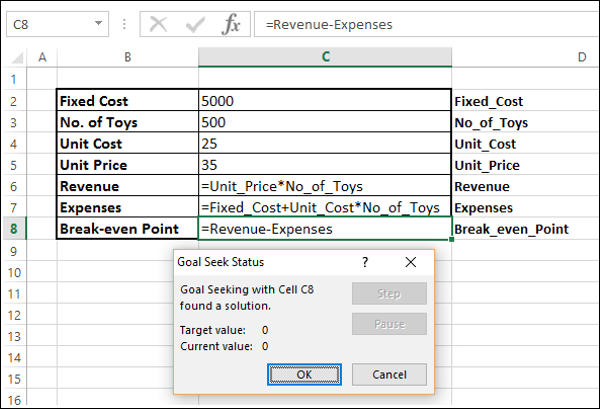# What-If Analysis with Goal Seek

Goal Seek is a What-If Analysis tool that helps you to find the input value that results in a target value that you want. Goal Seek requires a formula that uses the input value to give result in the target value. Then, by varying the input value in the formula, Goal Seek tries to arrive at a solution for the input value.

Goal Seek works only with one variable input value. If you have more than one input value to be determined, you have to use the Solver add-in. Refer to the chapter – Optimization with Excel Solver in this tutorial.

## Analysis with Goal Seek

Suppose you want to take a loan of 5,000,000 and you want to repay in 25 years. You can pay an EMI of 50000. You want to know at what interest rate you can borrow the loan.

You can use Goal Seek to find the interest rate at which you can borrow the loan as follows −

Step 1 − Set up the Excel cells for Goal Seek as given below.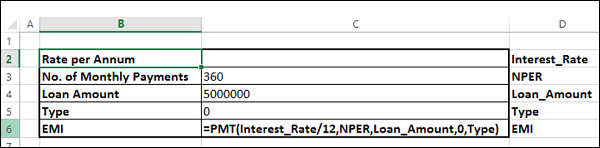Step 2 − Enter the values in column C corresponding to column D. The cell Interest_Rate is kept empty, as you have to retrieve that value. Further, though you know the EMI that you can pay (50000), that value is not included as you have to use the Excel PMT function to arrive at it. Goal Seek requires a formula to find the result. The PMT function is placed in the cell EMI so that it can be used by Goal Seek.

Excel computes the EMI with the PMT function. The table now looks like −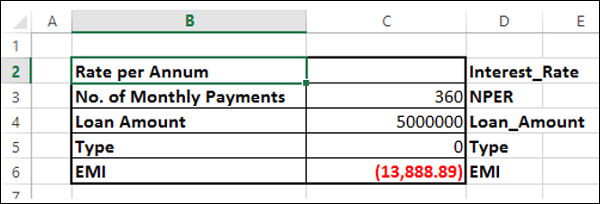As the Interest_Rate cell is empty, Excel takes that value as 0 and calculates the EMI. You can ignore the result -13,888.89.

Perform the Analysis with Goal Seek as follows −

Step 1 − Go to DATA > What If Analysis > Goal Seek on the Ribbon.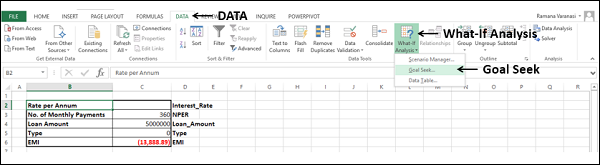The Goal Seek dialog box appears.

Step 2 − Type EMI in the Set cell box. This box is the reference for the cell that contains the formula that you want to resolve, in this case the PMT function. It is the cell C6, which you named as EMI.

Step 3 − Type -50000 in the To value box. Here, you get the formula result, in this case, the EMI that you want to pay. The number is negative because it represents a payment.

Step 4 − Type Interest_Rate in the By changing cell box. This box has the reference of the cell that contains the value you want to adjust, in this case the interest rate. It is cell C2, which you named as Interest_Rate.

Step 5 − This cell that Goal Seek changes, must be referenced by the formula in the cell that you specified in the Set cell box. Click OK.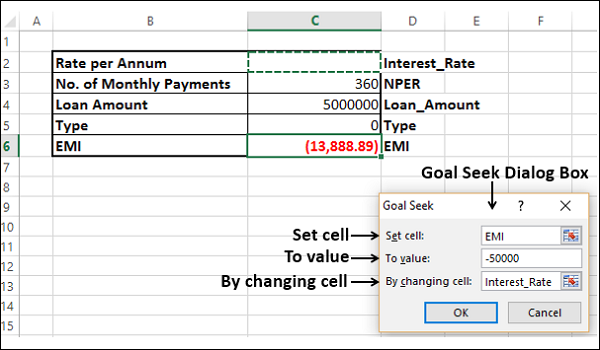Goal Seek produces a result, as shown below −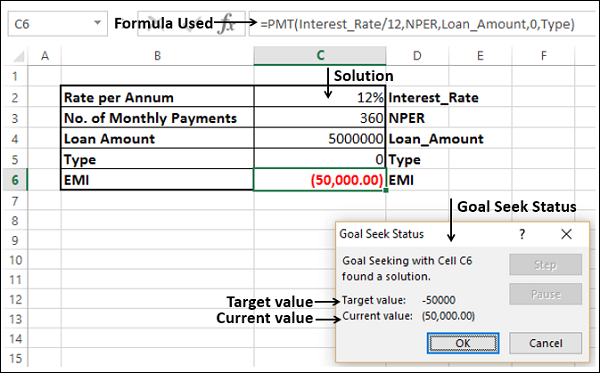As you can observe, Goal Seek found the solution using cell C6 (containing the formula) as 12% that is displayed in the cell C2, which is the interest rate. Click OK.

## Solving Story Problems

You can solve story problems easily with Goal Seek. Let us understand this with the help of an example.

### Example

Suppose there is a bookstore that has 100 books in storage. The original price of the book is 250 and certain number of books was sold at that price. Later, the bookstore announced a 10% discount on that book and cleared off the stock. You might want to know how many books are sold at the original price to obtain a total revenue of 24,500.

You can use Goal Seek to find the solution. Follow the steps given below −

Step 1 − Set the worksheet as given below.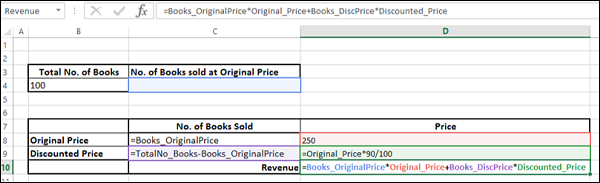Step 2 − Go to DATA > What If Analysis > Goal Seek on the Ribbon.

The Goal Seek dialog box appears.

Step 3 − Type Revenue, 24500 and Books_OriginalPrice in the Set cell box, To Value box and By changing cell box respectively. Click OK.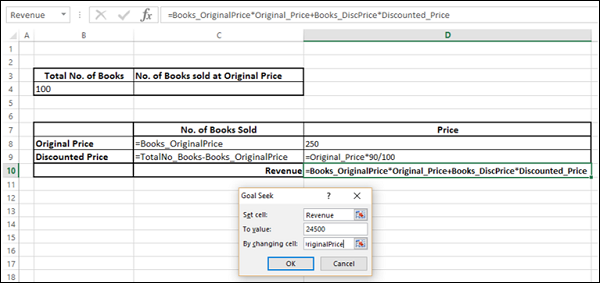Goal Seek displays the status and solution.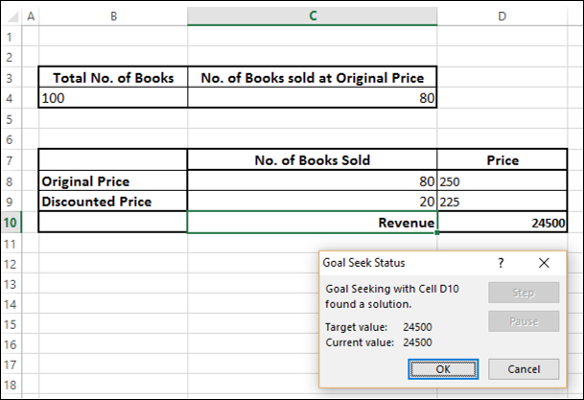If 80 books were sold at the original price, the revenue would be 24500.

## Performing a Break-even Analysis

In economy, break-even point is the point at which there is neither profit nor loss. This would mean −

Revenue = Expenses, or

Revenue – Expenses = 0

You can do break-even analysis with Goal Seek in Excel.

### Example

Suppose there is a store that sells toys. You might want to make a break-even analysis of the store. Collect the following information from the store −

• Fixed cost of the store.
• Unit cost of the toy.
• Number of toys to be sold.

You need to find at which price they should sell the toys to break even.

Step 1 − Set the worksheet as given below.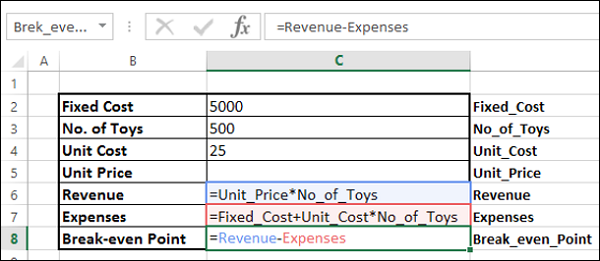Step 2 − Go to DATA > What If Analysis > Goal Seek on the Ribbon. The Goal Seek dialog box appears.

Step 3 − Type Break_even_Point, 0, and Unit_Price in the Set cell box, To value box and By changing cell box respectively. Click OK.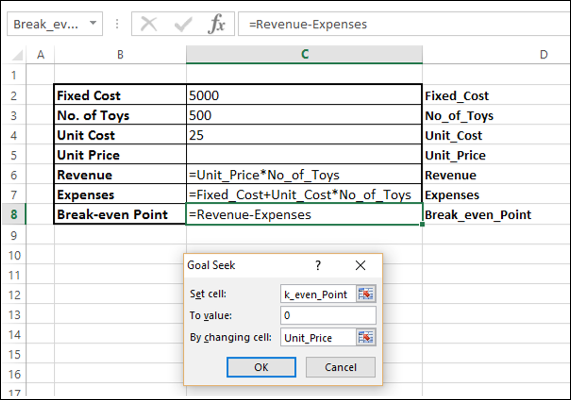As you can observe, Goal Seek gave the result that if the Unit Price is 35, the store will break even.